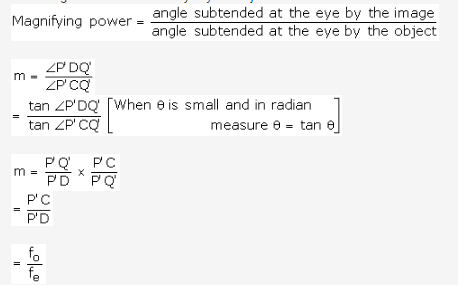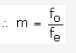# Draw a labelled ray diagram of an astronomical telescope

Draw a labelled ray diagram of an astronomical telescope. Write mathematical expression for its magnifying power.

Astronomical Telescope

It consists of two convex lenses called objective and eyepiece. The objective is of large focal length whereas the eyepiece is of short focal length. The distance between the two lenses can be adjusted by adjusting the tube which holds the lens.

The ray diagram showing the principle of the astronomical telescope is given below.
Magnifying power of an astronomical telescope may be defined as the ratio of the angle subtended at the eye by the image to the angle subtended at the eye by the object.From the ray diagram we know that:
PlC is the focal length of the objective and
PlD is the focal length of the eye piece.